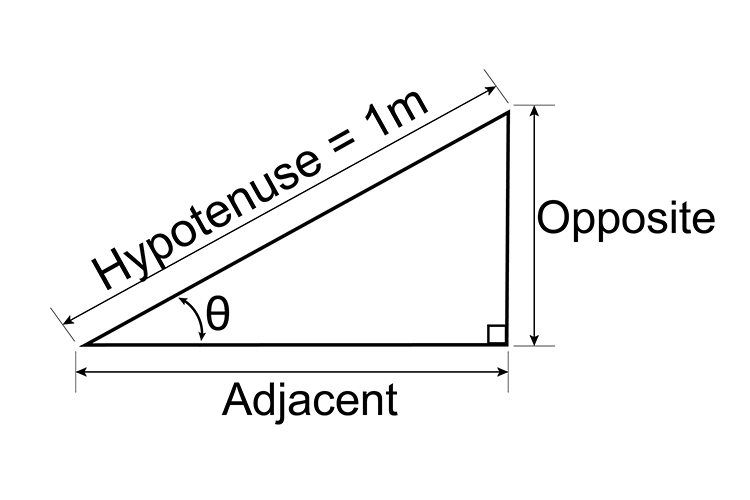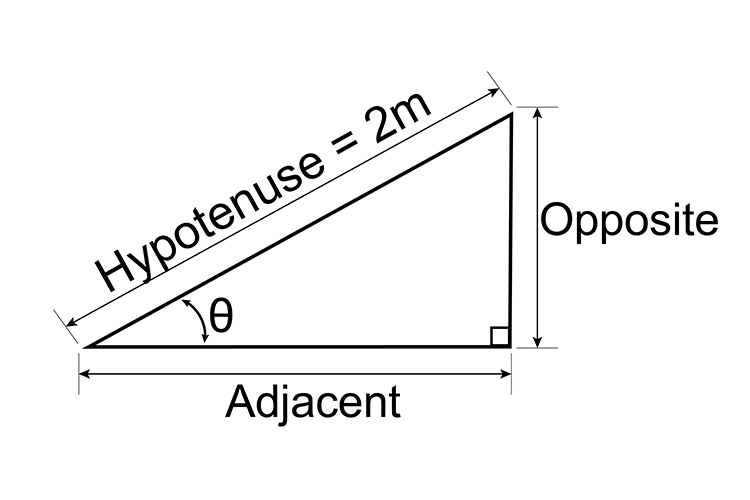# Sine examples

Example 1If the hypotenuse is 1 metre long and theta=35^@, how long is the opposite side?

Look up on the table/chart of sine (angle) for 35^@or look up sine 35^@ on a calculator.

 Angle Opposite distance (or sine) 35^@ 0.5736 metres

Hypotenuse = 1 metre,

theta=35^@,

Therefore, Opposite = 0.5736 metres.

Example 2

If the hypotenuse is 1 metre long and theta=45^@, how long is the opposite side?Look up on the table/chart of sine (angle) for 45^@ or look up sine 45^@ on a calculator.

 Angle Opposite distance (or sine) 45^@ 0.7071 metres

Hypotenuse = 1 metre,

theta=45^@,

Therefore, Opposite = 0.7071 metres.

Example 3

If the hypotenuse is 1 metre long, and the opposite side is 0.8 metres long, what is the angle theta  ?Look up on the table/chart of sine (angle) for 0.8 metres and find the angle.

 Angle Opposite distance (or sine) ? 0.8 metres

The nearest = 54° = 0.809 metres.

But on a calculator if you put in 0.8 and press inverse sin (sin-1), this gives you 53.13° (which is more accurate).

Example 4If the hypotenuse is 2 metres long and theta=45^@, how long is the opposite side?

Look up on the table/chart of sine (angle) for 45^@ or look up sine 45^@ on a calculator.

 Angle Opposite distance (or sine) 45^@ 0.7071 metres

But 0.7071 is for a 1m hypotenuse.

For a hypotenuse of 2m, the opposite would be twice as long.

Therefore 0.7071times2=1.4142

theta=45^@,